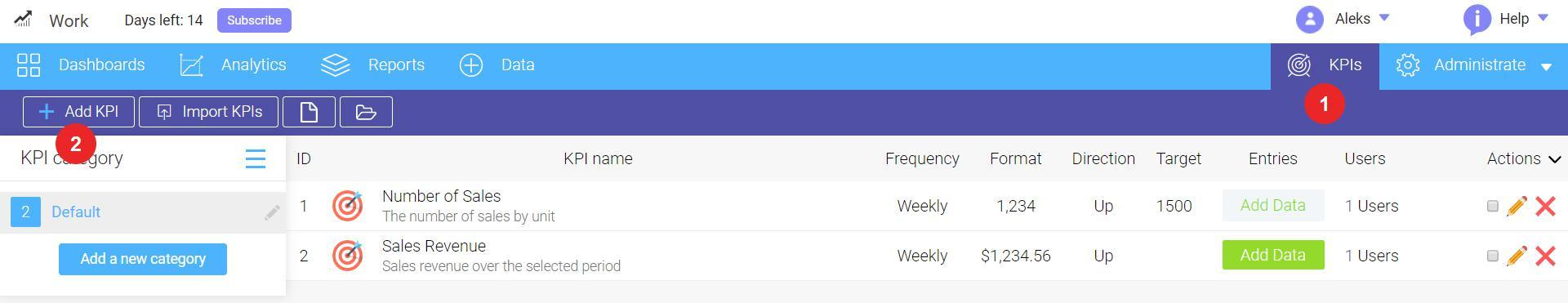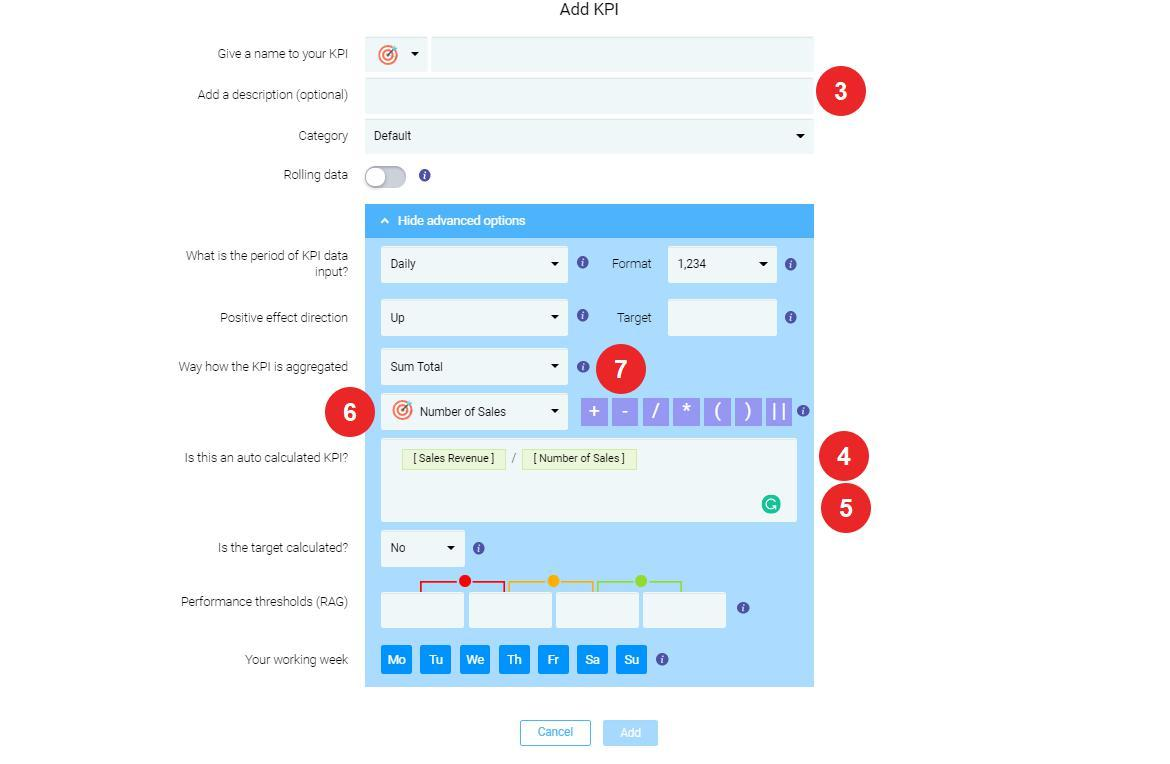# Calculated KPI's

Calculated KPIs is a useful tool to automate the calculation of numerical values for KPIs, such as ratios, averages,
minimum, maximum, etc.

Let us take an example. If you need to find the average sales value of a single product, you should analyze two KPIs:

• Number of Sales
• Sales Revenue

To calculate an average sales number per product, create a calculated KPI which will divide Sales Revenue by Number of Sales.

To create a calculated KPI. follow these steps:1. Go to KPIs tab;3. Enter the details (Name, target KPIs, etc.) for this Calculated KPI and then click Advanced Options;
4. Create the formula by selecting the KPI that will be used in calculations;
5. Choose an operator (in our case, we should take "/" - divide operator);
6. Select the KPI you want to divide by (denominator);
7. Choose how you want to aggregate the KPI: Sum Total (sum all values together) or Average (find a mathematical
average of all values);